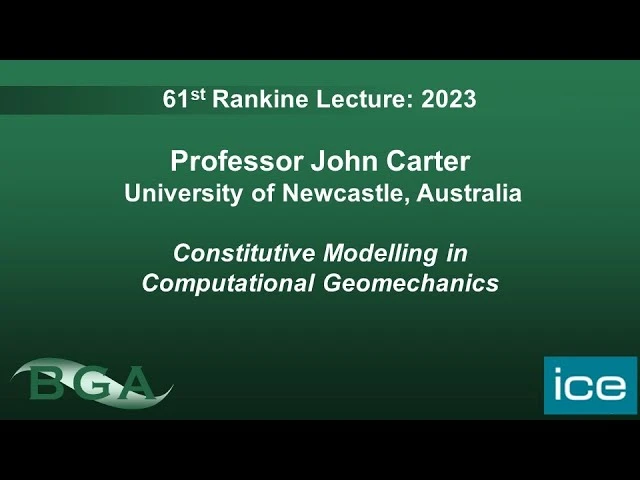# 61st Rankine Lecture: constitutive modelling in computational geomechanics61st Rankine Lecture (from: British Geotechnical Association Rankine Lecture)

The 61st Rankine Lecture took place on Wednesday, March 15, at Imperial College London.

Emeritus professor John Carter, of the University of Newcastle, Australia presented the lecture this year, which was titled “Constitutive modelling in computational geomechanics”.

It was stated at the beginning of the lecture how “all models are simply idealizations of the real world” and that “the kind of model we need to adopt, and its degree of sophistication are dependent of the questions being asked”.

The British Statistician, George E.P. Box was quoted as how “All models are wrong, but some are useful”, among others.

Professor Carter described constitutive models for soil as generally being “Mathematical relations between components of stress and strain” and how “In computational geomechanics, we generally seek mathematical solutions to what are known as boundary value problems or initial value problems. And very often in doing so, we would represent our soil body as a continuum. And it's useful to remind ourselves that these boundary value problems are usually formulated as a set of differential equations which might be subjected to particular conditions. These equations would set out in mathematical detail the three conditions of equilibrium, compatibility, and the constitutive relationships that have to be obeyed.”

The lecture considered mainly classical elastoplastic models.

As for some limitations, consideration was only given on single phase or saturated soils, mostly statically applied loading and, monotonic and some cyclic loading.

Some of the factors that complicate constitutive modelling of soils were also identified in the lecture, namely as:

-The non-linear relation between stress and strain

-Behaviour of natural soils in situ not same as reconstituted laboratory samples

-Rate effects, such as strain rate dependence and creep